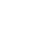M. Zuriqat, The homo separation analysis method for solving the partial differential equation, Italian Journal of Pure and Applied Mathematics, 40 (2018), 535-543.
In this work,the homo separation analysis method (HSAM) is applied to obtain the exact solution for linear and nonlinear partial differential equation. The proposed algorithm presents a procedure of constructing the set of base functions and gives the one-order deformation equation in a simple form. This analytical method is a combination of the homotopy analysis method (HAM) with the separation of variables method. The exact solution is constructed by choosing an initial guess in addition to only one term of the series obtained by HAM. This work veri es the validity and the potential of the HSAM for the study of nonlinear partial differential equation.
Publishing Year